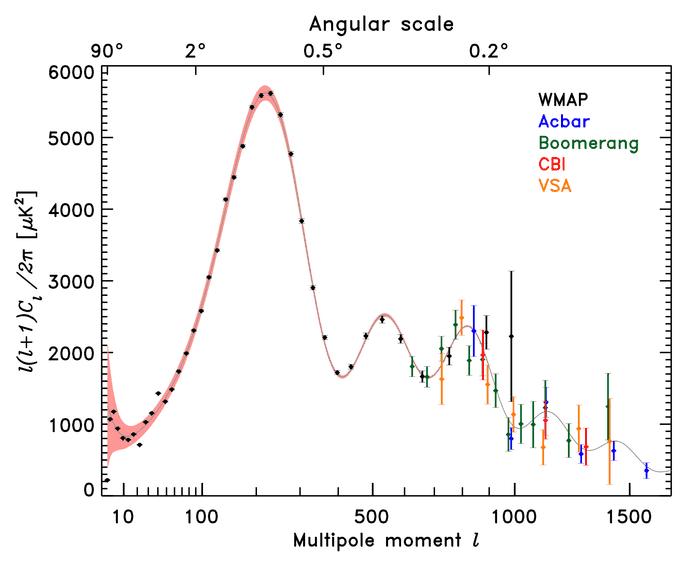# Understand CMB Anisotropies & Interpret Figures

• ChrisVer
In summary, the C_l^{TT} parameter in the given figure provides information on the amplitude of temperature fluctuations. The overall downward trend is due to the blurriness of the surface of last scattering, caused by the transition of primordial plasma to gas. The first peak represents the sound horizon, while the difference in even and odd peaks is due to the presence of dark matter. The ratio of odd-to-even peaks is a sensitive measurement of the ratio of normal matter to dark matter. The use of multipole index is because the sky has the geometry of a sphere, and the power spectrum C_\ell is the variance of all waves on the sky with a wavelength of approximately \pi/\ell radians. Further discussion on the topic can be

#### ChrisVer

Gold Member
Can someone help me understand what information we extract from such kind of figure?In fact the $C_l^{TT}$ parameter gives us information on the amplitude of the temperature fluctuations $\Delta T/T$... However I don't understand why there is such a peak formation (1 very large, 2 smaller and 2 even smaller), or why this is given in terms of multipole index $l$.

Some of the primary features on this graph:
1. The overall downward trend after the first peak is due to the fact that the primordial plasma took hundreds of thousands of years to transition to a gas. This has the impact of making the surface of last scattering (where the CMB photons came from) blurry. That blurriness is suppresses power at small angular scales. If it were not for this blurring effect, the CMB power spectrum would have very little overall increasing or decreasing trend.
2. The first peak is the "sound horizon". This is the distance that sound waves in the primordial plasma were able to travel from the time inflation ended to the time the CMB was emitted.
3. The difference in the even and odd peaks is due to dark matter. Within the primordial plasma, normal matter was able to bounce back out of gravity wells, while dark matter would just fall in. The first peak represents matter that just had enough time to fall into a gravitational potential well. The second peak is matter that had enough time to fall in and bounce back out. The third peak is matter that fell in, bounced out, then fell back in again. Normal matter contributes to all of the peaks, while dark matter only contributes to the odd-numbered peaks. This ratio of odd-to-even peaks is the most sensitive measurement we have of the ratio of normal matter to dark matter.

There are other things we can glean from the CMB power spectrum, but hopefully you can see why there is the overall trend here.

As for while it's given in terms of multipole index, this is because the sky has the geometry of the surface of a sphere, and the equivalent to Fourier transforms on the surface of a sphere are Spherical Hermonic transforms. In spherical harmonics, the power spectrum $C_\ell$ is the variance of all waves on the sky which have a wavelength on the sky of approximately $\pi/\ell$ radians across the surface of the sky.

•marcus